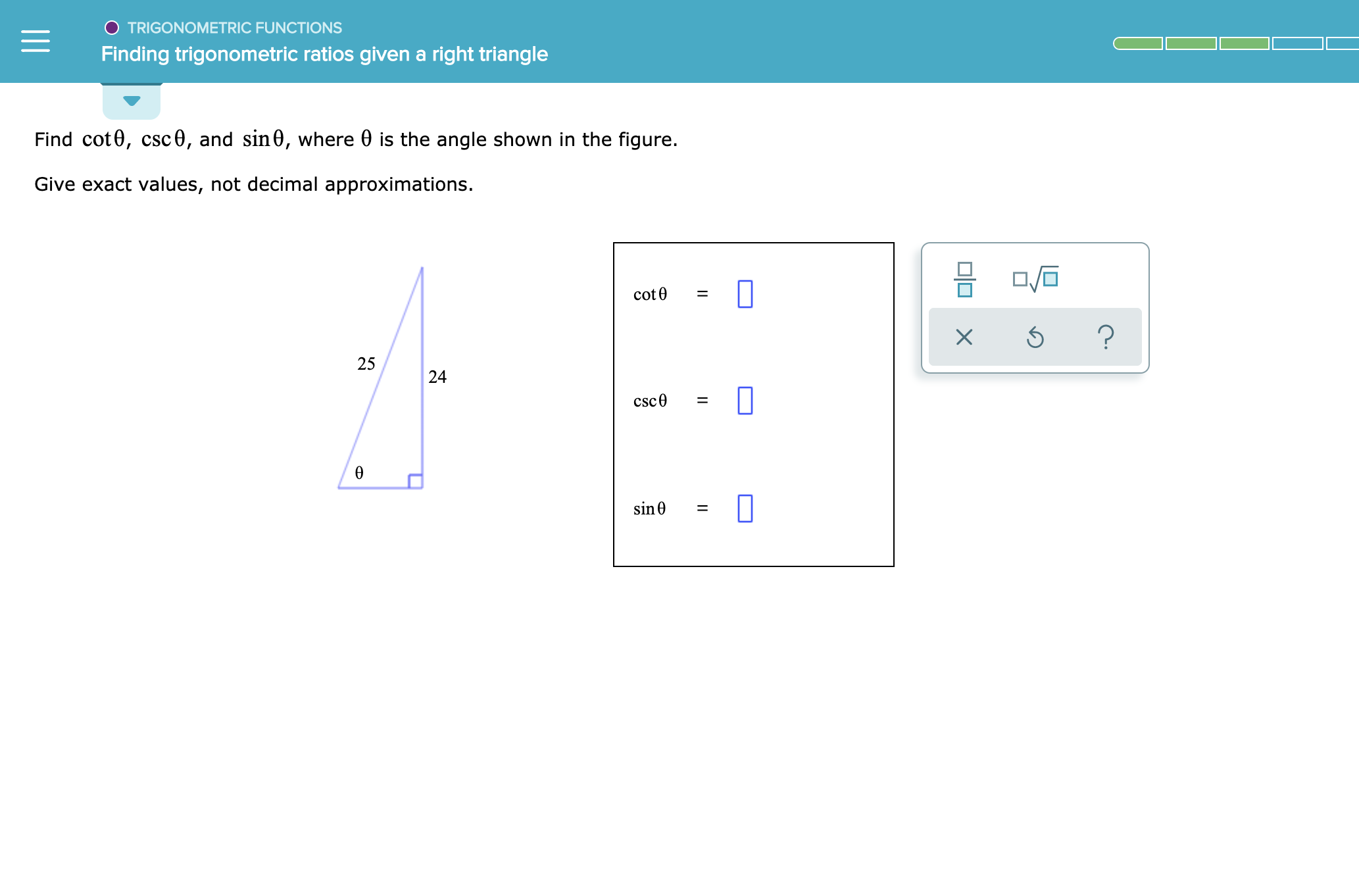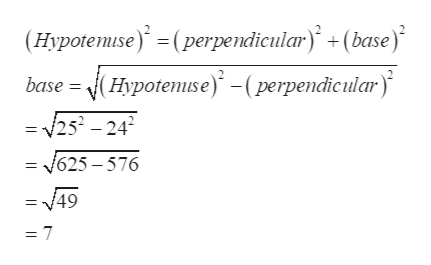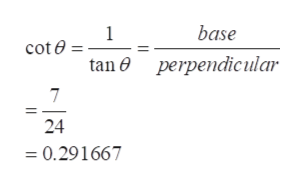TRIGONOMETRIC FUNCTIONSFinding trigonometric ratios given a right triangleFind cot0, csc0, and sin0, where 0 is the angle shown in the figure.Give exact values, not decimal approximations.cot=25csc0sin=24L

Question

see attachmenthelp_outlineImage TranscriptioncloseTRIGONOMETRIC FUNCTIONS Finding trigonometric ratios given a right triangle Find cot0, csc0, and sin0, where 0 is the angle shown in the figure. Give exact values, not decimal approximations. cot = 25 csc0 sin = 24 L fullscreen
Step 1

Refer to the question . for the given figure we have to find the cot theta, csc theta and sin theta.

Step 2

Use Pythagorean theorem to find the base of the triangle.help_outlineImage Transcriptionclose(Нурotemise)* %3 ( perpendicular)* - (base)* base - Hурotemse)* - ( рerpendicular)" = v252 -242 3D у625—576 49 = 7 fullscreen
Step 3

Use the cot formula to fin...help_outlineImage Transcriptionclosebase 1 cot e tan perpendicular 7 24 =0.291667 fullscreen

Want to see the full answer?

See Solution

Want to see this answer and more?

Our solutions are written by experts, many with advanced degrees, and available 24/7

See Solution
Tagged in

Other